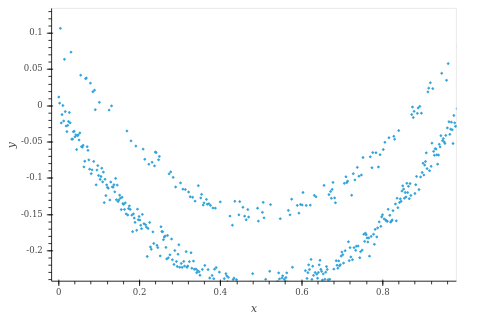# Mixture Model with Regression

Hi,
how can I fit data like this:This data was created with:

``````N = 500
xs = torch.linspace(0, 1, N)

eps = sample('_', dist.Normal(0, 0.01).expand((N, )))
inds = torch.randperm(N)[:N//4]
eps[inds] += 0.1
ys = xs*(xs - 1) + eps
``````

I tried applying the Mixture Model Tutorial and used this model:

``````from matplotlib import pyplot
from torch import tensor as t
import torch
import pyro
from pyro import sample, distributions as dist, plate, poutine
from pyro.infer import config_enumerate, SVI, TraceEnum_ELBO
from pyro.infer.autoguide import AutoDelta

@config_enumerate
def model(xs, ys):
a = sample('a', dist.Normal(0., 1.))
b = sample('b', dist.Normal(0., 1.))
weights = sample('weights', dist.Dirichlet(t([0.5, 0.5])))
scale = sample('scale', dist.LogNormal(0., 2.))

with plate('comp', 2):
c = sample('c', dist.Normal(0., 1.))

with plate('data', len(xs)):
assignment = sample('assignment', dist.Categorical(weights))
zs = a * xs**2 + b * xs + c[assignment]
pyro.sample('obs', dist.Normal(zs, scale), obs=ys)
return c

K = 2
def init_loc_fn(site):
if site["name"] == "weights":
# Initialize weights to uniform.
if site["name"] == "scale":
return (ys.var() / 2).sqrt()
if site["name"] == "c":
return data[torch.multinomial(torch.ones(len(xs)) / len(xs), K)]
raise ValueError(site["name"])

def initialize(seed):
global global_guide, svi
pyro.set_rng_seed(seed)
pyro.clear_param_store()
global_guide = AutoDelta(poutine.block(model, expose=['weights', 'locs', 'scale']),
init_loc_fn=init_loc_fn)
svi = SVI(model, global_guide, optim, loss=elbo)
return svi.loss(model, global_guide, xs, ys)

optim = pyro.optim.Adam({'lr': 0.01, 'betas': [0.8, 0.99]})
elbo = TraceEnum_ELBO(max_plate_nesting=1)
``````

But the training did not converge.

it’s hard to say but some things you might try:

• keep tweaking `init_loc_fn`
• lower learning rate and generally try different optimization settings, e.g. using `ClippedAdam`
• try HMC if you’re not wedded to SVI

Fitting the model with MCMC works now. But now I’m struggling with doing inference. So I did:

``````nuts = NUTS(model)
mcmc = MCMC(nuts, 400, warmup_steps=40, num_chains=1)
mcmc.run(xs, ys)
mcmc.summary()
``````

Which gives good results in my opinion:

``````                mean       std    median      5.0%     95.0%     n_eff     r_hat
a          1.02      0.01      1.02      1.00      1.04    154.75      1.00
b         -1.02      0.02     -1.02     -1.04     -0.99    151.97      1.00
weights      0.74      0.04      0.74      0.68      0.81     29.27      1.00
weights      0.26      0.04      0.26      0.19      0.32     29.27      1.00
scale          0.01      0.00      0.01      0.01      0.01    400.80      1.00
c         -0.00      0.00     -0.00     -0.01      0.00    180.90      1.00
c          0.10      0.00      0.10      0.09      0.11    197.50      1.00
``````

For inference I tried:

``````samples = mcmc.get_samples(1000)
pred = Predictive(model, samples)
assign_pred = pred(xs, ys)['assignment']
assign_pred.numpy().mean(axis=0)
``````

But this results in just one class for all samples. Is this the correct method or did I misunderstand something?

Help would be really appreciatedI don’t think HMC will work since it integrates out the discrete variable, whereas you are looking to infer its value given your observation.